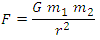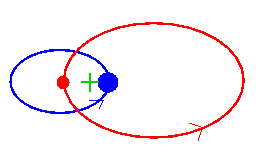## Saturday, 20 July 2013

### Gravity, Motion and Orbits explained by Classial Physics

Gravity is a attractive force. Classical physics defines gravity as an attractive force that exists between every matter in this universe. It is the force that defines Orbits, Free fall and black holes. Even the rings of Jupiter, Saturn, Neptune and Pluto was defined by gravity. The scientific study of gravity was started in 1590 by Galileo Galilei. In 1665-1666 Newton gave his Gravitational law.

Newton's law of gravitation

Newton's law of gravitation states that every body in this universe attracts every other body which is directly proportional to their masses and inversely proportional to the square of distance between them. If their masses is doubled force gets doubled and if their distance is doubled then the force of gravity becomes one-fourth.

i.e,  Mass of the bodies
and  1/r2 , where r is the distance between two bodies

Combining the two we get,where G is gravitational constant with value of G = 6.673 × 10 -11 m3/kg•s2.

Weight and Free fall

The size of gravitational force acting on an object is called its weight. The weight of a body changes according to its local gravity. For example, Weight of a 1 Kg object is 9.8 N on earth and 1.65 N on moon.

Free fall is any motion of a body where its weight is the only force acting upon it. Free fall is caused by gravity. Free fall is the phenomenon which is completely explained by classical physics.

Laws of motion

Newton, in 1686 or 1688, formulated his laws of motion which was a breakthrough in classical physics. Before laws of motion people believed that an object would be in motion only if it is acted upon by a force.

First law of motion

This law states that a body would continue to be at rest or in motion unless it is acted upon by an external net force that would either stop it or set it in motion or change its direction. This is examplified by the fact that you are thrown forward when a bus stops suddenly.

Second law of motion

The acceleration of a body is directly proportional to, and in the same direction as, the net force acting on the body, and inversely proportional to its mass. Thus, F = ma, where F is the net force acting on the object, m is the mass of the object and a is the acceleration of the object. If you apply equal force on two objects of different mass then the object with greater mass would have greater momentum (i.e capacity to be in motion).

Third law of motion

When one body exerts a force on a second body, the second body simultaneously exerts a force equal in magnitude and opposite in direction to that of the first body.

Orbits

Every planet follow a path around the Sun called orbits. The shapes of these orbits are primarily governed by Kepler's law.

Kepler's laws are:

• The orbit of every planet is an ellipse with the Sun at one of the two foci.
• A line joining a planet and the Sun sweeps out equal areas during equal intervals of time.
• The square of the orbital period of a planet is proportional to the cube of the semi-major axis of its orbit.Illustration of second law. Courtesy : Wikipedia

When one object is in orbit around another of higher mass, it is in free fall toward the larger body. It experiences a constant gravitational acceleration toward the larger object that deflects what would otherwise be its straight line motion into a curved trajectory. Many closed orbits are elliptical in nature. Earth, moon and many other celestial bodies have circular path but Pluto and Neptune have eccentric, highly elongated orbits. The reason behind elliptical orbit of Pluto and other celestial bodies was not explained by classical physics. It was the later explained by Einstein's Gravity.

Common center of mass

In an orbital system of two bodies, the smaller bodies doesn't simply orbit the larger body but both the bodies orbit around their common center of mass. For the Earth-moon system, it is located deep inside the earth. This is why Moon follow a elliptical orbit path around earth. The orbit of the Earth is not observed.Common center of mass

Watch the following video for understanding laws of motion :

<- Previous Chapter                                                                                                                 Next Chapter ->